## Sylvester's Sequence

The sequence defined byand the Recurrence Relation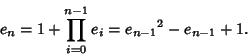(1)

This sequence arises in Euclid's proof that there are an Infinite number of Primes. The proof proceeds by constructing a sequence of Primes using the Recurrence Relation(2)

(Vardi 1991). Amazingly, there is a constant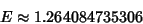(3)

such that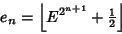(4)

(Vardi 1991, Graham et al. 1994). The first few numbers in Sylvester's sequence are 2, 3, 7, 43, 1807, 3263443, 10650056950807, ... (Sloane's A000058). The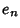satisfy(5)

In addition, ifis an Irrational Number, then theth term of an infinite sum of unit fractions used to representas computed using the Greedy Algorithm must be smaller than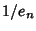.

Theof the first few Primeare 0, 1, 2, 3, 5, .... Vardi (1991) gives a lists of factors less thanoffor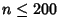and shows thatis Composite for. Furthermore, all numbers less thanin Sylvester's sequence are Squarefree, and no Squareful numbers in this sequence are known (Vardi 1991).

References

Graham, R. L.; Knuth, D. E.; and Patashnik, O. Research problem 4.65 in Concrete Mathematics: A Foundation for Computer Science, 2nd ed. Reading, MA: Addison-Wesley, 1994.

Sloane, N. J. A. Sequence A000058/M0865 in An On-Line Version of the Encyclopedia of Integer Sequences.'' http://www.research.att.com/~njas/sequences/eisonline.html and Sloane, N. J. A. and Plouffe, S. The Encyclopedia of Integer Sequences. San Diego: Academic Press, 1995.

Vardi, I. Are All Euclid Numbers Squarefree?'' and PowerMod to the Rescue.'' §5.1 and 5.2 in Computational Recreations in Mathematica. Reading, MA: Addison-Wesley, pp. 82-89, 1991.